Univerza na Primorskem Fakulteta za matematiko, naravoslovje in informacijske tehnologije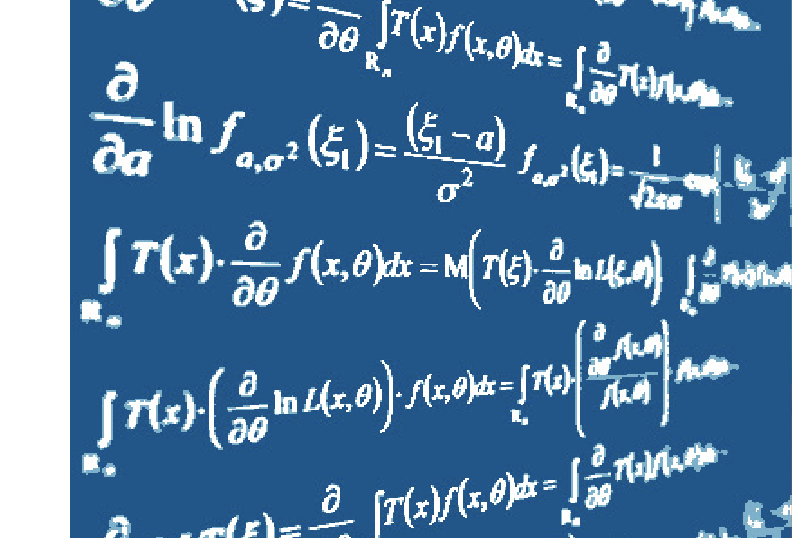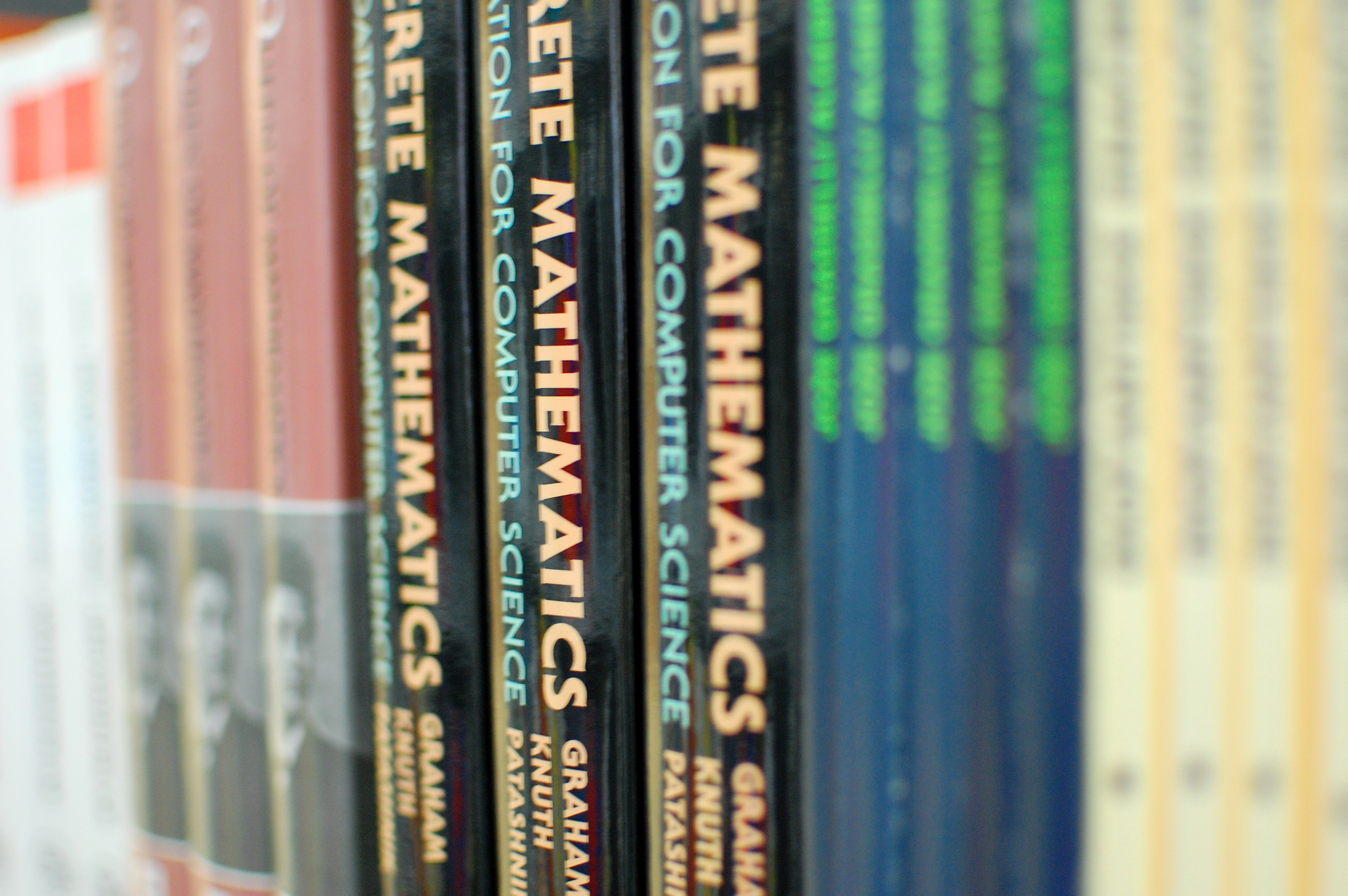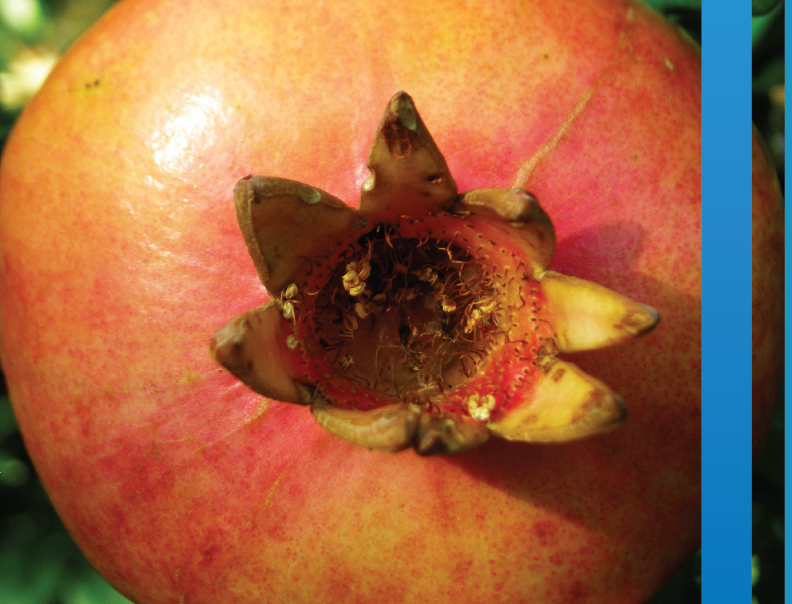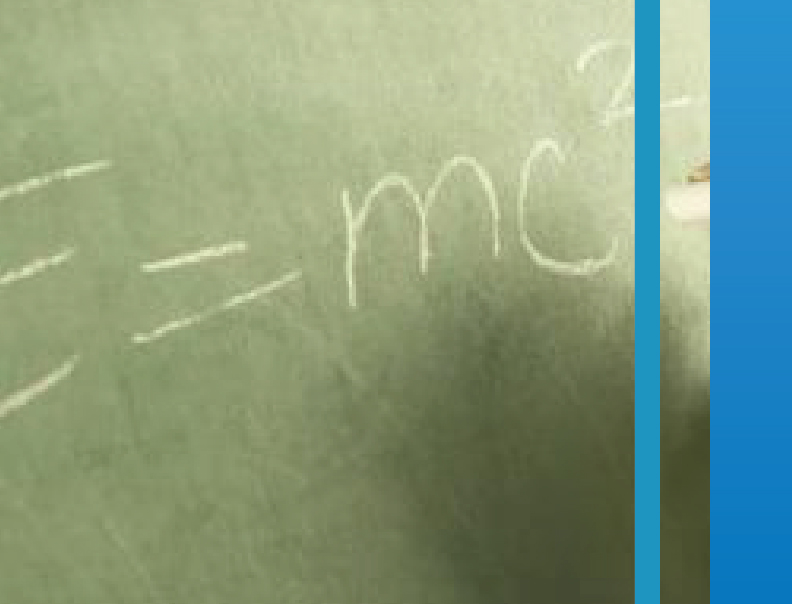SI | EN# Raziskovalni matematični seminar - Arhiv

 2020 2019 2018 2017 2016 2015 2014 2013 2012 2011 2010
 1 2 3 4 5 6 7 8 9 10 11 12
Datum in ura / Date and time: 30.5.12
(13:45-14:30)
Predavatelj / Lecturer: Karla Počkaj
Naslov / Title: Construction of rigid body motions
Vsebina / Abstract:

In this talk I will present the rigid body motions and the interpolation of given positions, e.g. points and orientations of a moving object.

The solution of this problem is required in Computer Graphics in order to animate objects, as well as in Robotics, e.g. for path planning of robot manipulators. Because of their fast and stable algorithms, the methods which interpolate rotations using quaternions seem to become very popular. The results on the spherical rational spline motions are extended to the spatial ones, by combining them with rational trajectories of the origin. An interpolation scheme based on cubic spline functions will be discussed since it possesses some features which make it a suitable tool in many industrial applications.

Datum in ura / Date and time: 30.5.12
(3:00-13:45)
Predavatelj / Lecturer: Boštjan Frelih
Naslov / Title: Amply regular graph products
Vsebina / Abstract:

A regular graph is called amply regular if the number of common neighbours of two adjacent vertices is independent of the choice of these two vertices and the number of common neighbours of
two vertices at a distance 2 is independent of the choice of these two
vertices. In this talk we present some results about amply regular
lexicographic, deleted lexicographic and co-normal graph products.

Datum in ura / Date and time: 28.5.12
(11:00-12:00)
Predavatelj / Lecturer: dr. Fabio Vlacci (Università degli Studi di Firenze, Italy)
Naslov / Title: Introduction to basic properties of a new class of regular functions of (hypercomplex) quaternionic variable
Vsebina / Abstract:

The aim of this talk is to give a self-contained introduction to a new theory of regular functions over quaternions and, more in general, over a non commutative algebra. In particular, we'll give a (historic) overview of different possible approaches to a definition of regularity for quaternionic functions and focus our  attention on the geometric/analytic properties related to the class of functions which are regular according to the recent definition given by Gentili & Struppa in 2007.

We'll also present some applications and results which are in some cases a generalization of the similar results in Complex Analysis, in others are quite unexpected and promising for the study of new phenomena. Some related topics will be then considered in order to show what kind of  difficulties or open problems are still under investigation.

Datum in ura / Date and time: 28.5.12
(10:00-11:00)
Predavatelj / Lecturer: dr. Wilfried Imrich (University of Leoben, Austria)
Naslov / Title: The distinguishing and endomorphism distinguishing number of graphs and groups
Vsebina / Abstract:

The distinguishing number of graphs was introduced by Albertson and Collins 1996, and has spawned a wealth of results on finite and infinite structures. The idea of the distinguishing number is to break symmetries efficiently, where "symmetries" stands or automorphisms. If one also wishes to break endomorphisms, one arrives at the endomorphism distinguishing number. Although endomorphisms are quite untractable, compared to automorphisms, many interesting results for finite and infinite structures immediately generalize from automorphisms to endomorphisms, and many new and interesting problems arise.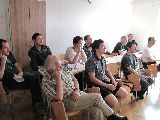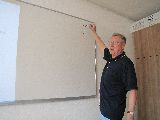Datum in ura / Date and time: 24.5.12
(13:00-13:45)
Predavatelj / Lecturer: Hiroki Koike
Naslov / Title: The isomorphism problem for cyclic configurations
Vsebina / Abstract:

Abstract: An incidence geometry consists on a set of points X of points and a set of blocks, or lines (subsets of X), such that two blocks intesect in at most one point. An incidence geometry is a *configuration* if every line has the same number of points and every point is incident with the same number of lines. A configuration is *cyclic* if its automorphism group contains a regular cyclic subgroup. The isomorphism problem for cyclic configurations asks for sufficient conditions to check wether two cyclic configurations are isomorphic or not. We say that two cyclic configurations are *multiplier equivalent* if some element of the multiplicative group of Z_n induces an isomorphism. A related problem is that for which n two isomorphic cyclic configurations  are multiplier equivalent. In this talk we show some known results for general cyclic combinatorial objects and we present some partial results for the latter problem.

Datum in ura / Date and time: 24.5.12
(13:45-14:30)
Predavalnica / Location: Samed Bajrić
Naslov / Title: The Representations of Vectorial Boolean Functions

Datum in ura / Date and time: 21.5.12
Predavatelj / Lecturer: dr.Ilya Ponomarenko (V. A. Steklov Institute of Mathematics,Russia)
Naslov / Title: Solvability of finite Schur groups
Vsebina / Abstract:

Let G be a Schur group, i.e. each Schur ring over G arises from
a suitable permutation group which contains a regular subgroup isomorphic
to G. Then the group G is solvable. This is a joint work with A.Vasiliev.

Datum in ura / Date and time: 17.5.12
Predavatelj / Lecturer: Alexander Vasilyev
Naslov / Title: Approach of lazy calculations in application to topological graph indices in Sage. Introduction to a new molecular class
Vsebina / Abstract:

Abstract: Previously we introduced a molecular graph class based on standard Sage graph class. The classical approach of object-oriented model proved to be not at its best on a huge amount of data. The conception of lazy calculations and some optimizations allowed us to dramatically increase the performance. We will talk about the nature of such problems in calculations and some solutions to avoid them.

Datum in ura / Date and time: 17.5.12
Predavatelj / Lecturer: Iva Antončič
Naslov / Title: Half-arc-transitive graphs of particular orders
Vsebina / Abstract:

Abstract: A graph is said to be half-arc-transitive if the automorphism group of the graph acts transitively on the vertex set and the edge set of the graph, but not on the arc set of the graph. In this talk we present results on classification of half-arc-transitive graphs of orders p, 2p, 3p, 4p, pq, 2pq, p^2, p^3, p^4 and 2p^2, where p and q are primes, with an emphasis on tetravalent graphs.

Datum in ura / Date and time: 14.5.12
Predavatelj / Lecturer: dr. Roman Nedela (Matej Bel University, Slovakia)
Naslov / Title: 6-decompositions of snarks
Vsebina / Abstract:

A snark is a cubic graph with no proper $3$-edge-colouring. In 1996, Nedela and \v Skoviera proved the following theorem:

Let $G$ be a snark with an $k$-edge-cut, $k\geq 2$, whose removal leaves two
$3$-edge-colourable components $M$ and $N$. Then both $M$ and $N$ can be completed to two snarks $\tilde M$ and $\tilde N$ of order not exceeding that of $G$ by adding at most $\kappa(k)$ vertices, where the number $\kappa(k)$ only depends on $k$. The known values of the function $\kappa(k)$ are $\kappa(2)=0$, $\kappa(3)=1$, $\kappa(4)=2$ (Goldberg, 1981), and $\kappa(5)=5$ (Cameron, Chetwynd, Watkins, 1987). The value $\kappa(6)$ is not known and is apparently difficult to calculate. In 1979, Jaeger conjectured that there are no 7-cyclically-connected snarks. If this conjecture holds true, then $\kappa(6)$ is the last important value to determine. Our talk is aimed attacking the problem of determining $\kappa(6)$ by investigating the structure and colour properties of potential complements in $6$-decompositions of snarks. We find a set of $14$ complements that suffice to perform $6$-decompositions of snarks with at most $30$ vertices. We show that if this set is not complete to perform $6$-decompositions of all  snarks, then $\kappa(6)\geq 20$ and there are strong restrictions on the structure of (possibly) missing complements.
Part of the proofs are computer assisted.10.05.2012 Lecturer: Nina Chiarelli

Title: Split graphs and threshold graphs
Abstract: The chromatic number of a graph is the minimum number of colors needed to color the vertices of the graph in such a way that no adjacent vertices have the same color. A clique in a graph is a subset of pairwise adjacent vertices. Graphs for which the chromatic number
of every induced subgraph is equal to the size of its largest clique, are called perfect graphs. In this talk we will give basic definitions and present different characterizations (with forbidden induced subgraphs and with degree sequences) for split graphs and threshold graphs, two of the many families of perfect graphs.